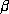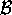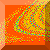# 5. Accessible and Inaccessible Basin Boundary Points

We say a basin boundary pointis accessible from a particular basin, if there is another point in the interior of the particular basin which can be connected toby a finite length curve that contains no boundary points except. We sayis inaccessible from a particular basin if this is not possible, and ifis inaccessible from every basin we say thatis inaccessible. (Accessible and inaccessible basin boundary points were first discussed in Grebogi et al. [1986, 1987c].) In this section we characterize the accessibility and inaccessibility of basin boundary points for our system. In particular, we show the following: (i) All basin boundary points that are accessible from the red region lie on the stable manifold of the fixed point (1/3,0) (and similarly for the blue region and the fixed point (0,0), and for the green region and the fixed point (2/3,0)). (ii) All basin boundary points not on the stable manifold of one of the three fixed points are inaccessible. (iii) The stable manifolds of the fixed points (0,0), (1/3,0), (2/3,0) are each dense in the basin boundary (i.e., every neighborhood of a basin boundary point contains part of the stable manifolds of all three fixed points, and the basin boundary is thus the closure of the stable manifold of any of the fixed points).

To proceed we introduce trapping regions for each of the three exits. Trapping regions are regions in the surface of section where all trajectories that exit the system will ultimately enter, and once they entered, the trajectories will not leave the trapping regions until they exit the scattering region (and hence the system). In order to find appropriate trapping regions, we look at how the trajectories leave the exits. We concentrate on one of the exits, say exit I, and the results will apply to the other two exits using symmetry arguments. We first note the three largest (red) connected regions in the basin of exit I (see Fig. 6); these three regions are shown schematically in Fig. 8 and are labeled A, B, and C. Any trajectory that leaves through exit I will bounce off either disk 1, 2, or 3 in its last collision within the scattering region. The orbit locations in the surface of section corresponding to the last collision in the scattering region before going through exit I are the three shaded areas in regions A, B, and C shown in Fig. 8. Specifically, the shaded area in region A is the set of initial conditions that starts from disk 1 and leaves directly through exit I with no additional collisions within the scattering region, while the shaded area in region B is the set that starts from disk 2 and immediately leaves through exit I, and the shaded area in region C is the set that starts from disk 3 and immediately leaves through exit I. The union of regions A, B, and C form what we called a trapping set for exit I. Every point in the trapping set eventually leaves through exit I (the trapping set is red in Fig. 6), and, more importantly, any point that leaves through exit I must eventually enter the trapping set. The latter follows since if a trajectory leaves through exit I, it must spend its last iterate in one of the shaded areas of Fig. 8, and these are located in the trapping set for exit I. Similar trapping sets exist for the other two exits (they can be seen in Fig. 6, where the three largest green swatches correspond to the trapping set for exit II, and the three largest blue swatches correspond to the trapping set for exit III.)

We now show that the boundaries of the trapping sets (aside from the vertical lines s = 0, 1/3, 2/3) lie on finite segments of the stable manifolds of the fixed points. While the ``sprinkler algorithm'' used earlier in the section generated stable and unstable manifolds of the invariant set, it does not generate stable and unstable manifolds for specific points within the invariant set. For a picture of the stable manifold of the fixed points, we employ an algorithm introduced in You et al.  which generates very accurate approximations of stable and unstable manifolds. Since the system we are studying has three-fold symmetry, we only need to compute the stable manifold for one of the fixed points. We computed stretches of the stable manifold corresponding to the fixed point (1/3,0) as shown in Fig. 9. From Fig. 9, we see two branches of the stable manifold of the fixed point (1/3,0), one coming towards the fixed point from the upper left and one coming towards the fixed point from the lower right. We observe from comparison of Figs. 8 and 9 that the upper left and lower right segments are boundaries of the regions A and B respectively. Portions of the two segments of the stable manifold immediately to the left and right of the fixed point (1/3,0) are then iterated backwards to obtain the segments bounding the top and bottom of region C. For clarity, segments of the stable manifold are shown either dotted or solid to indicate how they are iterated forward in time. For example, the top segment bounding region C (solid) gets mapped to the upper portion of the curve (solid) bounding region A and next into the middle segment bounding region B (solid). Similarly, the bottom segment bounding region C (dotted) gets mapped to the lower portion of the curve (dotted) bounding region B and next into the middle segment bounding region A (dotted). Thus the boundary of trapping set I lies on finite segments of the stable manifold of the fixed point (1/3,0).

In Fig. 10, we have superimposed the segment of an unstable manifold of the fixed point (1/3,0) computed using the algorithm of You et al.  over the color basin picture. It is clear that the manifold crosses all three basins. From this fact, the following argument of Kennedy & Yorke  shows that points on the stable manifold of (1/3,0) are Wada and are accessible from the red basin. Denote the three different basins by1,2and3, and let B1, B2and B3 be subsets corresponding to thei's. Suppose p is a saddle fixed point on the basin boundary, and the unstable manifold of p crosses portions of all the three different basins. Examining successive pre-images of the Bi's, we note that they approach the stable manifold of p because the distance between points on the unstable manifold contracts under the inverse map. Similarly, pre-images of the Bi's will expand along the direction of the stable manifold of p as shown in Fig. 11. Thus, successive pre-images of the Bi's get arbitrary close to the stable manifold of p, and every point on the stable manifold of p is on the boundary of the Bi's for each i [Kennedy & Yorke, 1991]. Thus, every point on the stable manifold of p is a Wada point. On the other hand, we emphasize that the boundaries of the trapping region for red are accessible from the red region by our choice of the trapping region.

Now consider an arbitrary boundary point. We consider two possibilities: Either (a) the boundary pointiterates to the boundary of a trapping region in a finite number m of iterates (i.e.,is on the stable manifold of the appropriate fixed point), or (b) it does not. In case (a) fm() is an accessible boundary point. Without loss of generality, suppose fm() is accessible from the red basin, then there is a curve in the red region connecting it to an arbitrarily nearby interior pointof the red region. Iterating fm(),and the curve connecting fm() andbackward m steps, we have a curve connectingto the interior point f-m(). Thusis accessible, and any point point accessible from red is on the stable manifold of (1/3,0). Also, since the boundary is Wada, any boundary point not accessible from the red basin has an interior red point arbitrarily nearby. Moving along a straight line from the interior red point toward the boundary point that is not accessible from red, we must encounter a first boundary point, and this is by definition a boundary point accessible from the red region. This red accessible boundary point is on the stable manifold of (1/3,0). Hence any boundary point inaccessible from red is visited arbitrarily closely by the stable manifold of (1/3,0); i.e., this stable manifold is dense in the basin boundary.

Now consider case (b). In this case the orbit from the boundary pointremains in the scattering region for all time but is not on the stable manifold of one of the fixed points. We note that, as shown in Fig. 12, the invariant set lies within the shaded regions bounded by fixed point stable and unstable segments. As shown in Fig. 12, for a sufficient number of iterates m, the point fm() will lie in the interior of one of the shaded regions. To see this, recall that iterates of the boundary points approach the invariant set. Thus they must either (i) come near the shaded region of Fig. 12 and stay near it without entering, (ii) stay on its boundary without entering, or (iii) enter it. Possibilities (i) and (ii) can be ruled out as follows. The orbit cannot fall on the fixed point stable manifold segments bounding the stable region since we are considering case (b). Also iterates ofcannot be close to and outside of the shaded region by being near these stable manifold segments, since they would then be in a trapping region andis a boundary point. On the other hand, consideration of the dynamics of the map shows that iterates of points on or sufficiently near the unstable manifold boundaries of the shaded region map to its interior. Thus the orbit fromenters the shaded region of Fig. 12.

Using the same construction and notation as in Sec. 4, we recall that iterates of the line segment L() contained within D() expand exponentially with time and align with the unstable manifold of the invariant set. Also, for large enough m, fm() will be in the interior of the shaded region of Fig. 12, and fm(L()) will be long enough that the upper right segment of fm(L()) starting from fm() and the lower left segment starting from fm() both cross segments of the fixed point stable manifolds bounding the shaded region in which fm() lies (see Fig. 12). These stable manifold segments are Wada (they are part of the trapping region boundaries) and hence possess red, blue, and green striped regions accumulating on them. Thus iterating backwards to the original boundary point, we see that there are red, blue, and green striped regions in D() on both sides of. Sincecan be arbitrarily small, red, blue, and green stripes are arbitrarily close toon both sides of. Henceis inaccessible, since any curve from an interior point drawn tomust always cross such stripes (in fact, it must cross an infinite number of stripes).Chaos at Maryland Home Page